##### Tags
Spice Projects eBook Simulation eBook Simulation
Recommend Projects

# EasyEDA functions

5 years ago 10276
• Description
• Documents
• BOM
• Attachments
• Members

## Description

Examples of the various functions available in EasyEDA.

Some of the functions are built-in functions of ngspice. Some are EasyEDA implementations of new functions or are functions that that improve on the ngspice versions in some way, such as improving convergence, are more reliable in simulation, are more widely applicable in expressions and B sources or are just easier to use.

Note that, unless otherwise stated, functions used in expressions that are in turn used to define component values, in .param statements and in .func function definitions in EasyEDA operate on - and return - only real values.

This project is part of the EasyEDA Simulation eBook.

## limit(x, L, U)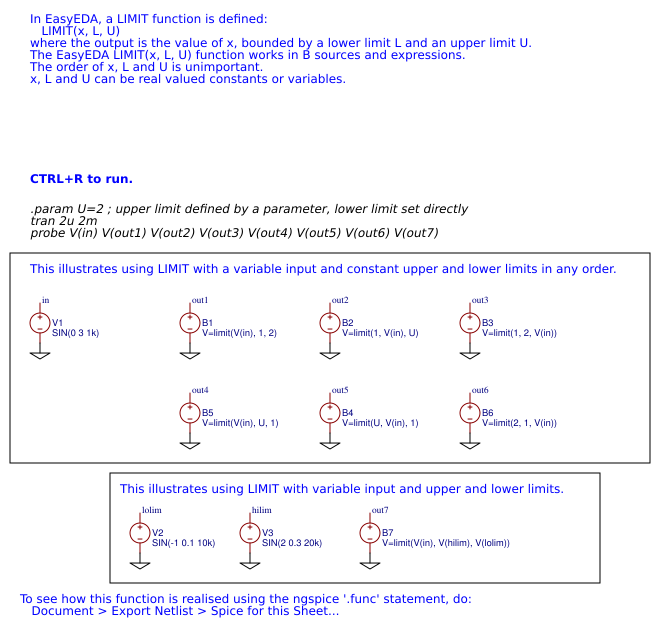## pow(x,a), pwr(x,a), pwrs(x,a) power functions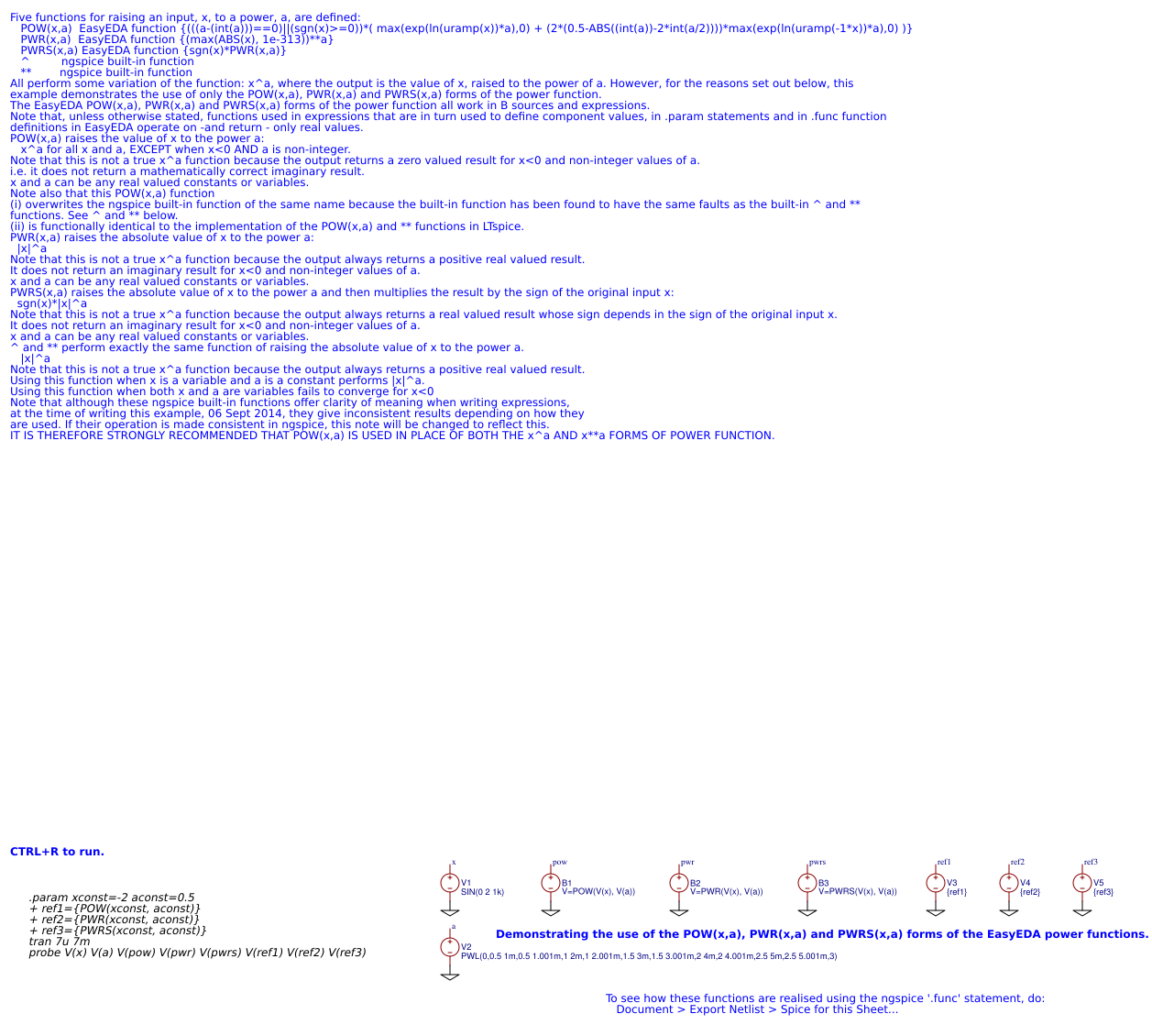## int(x)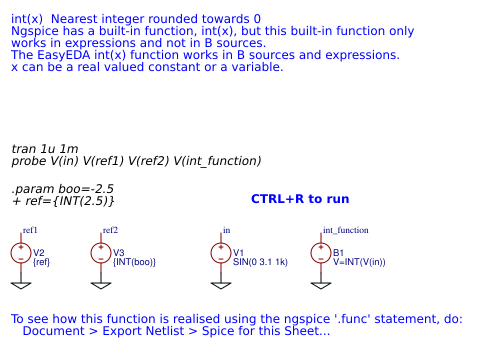## uramp(x)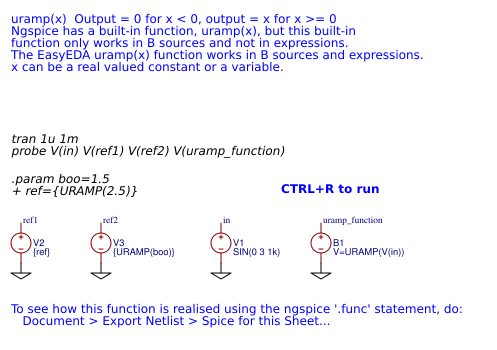## max(x,y)## min(x,y)## floor(x)## ceil(x)## abs(x)## sqr(x)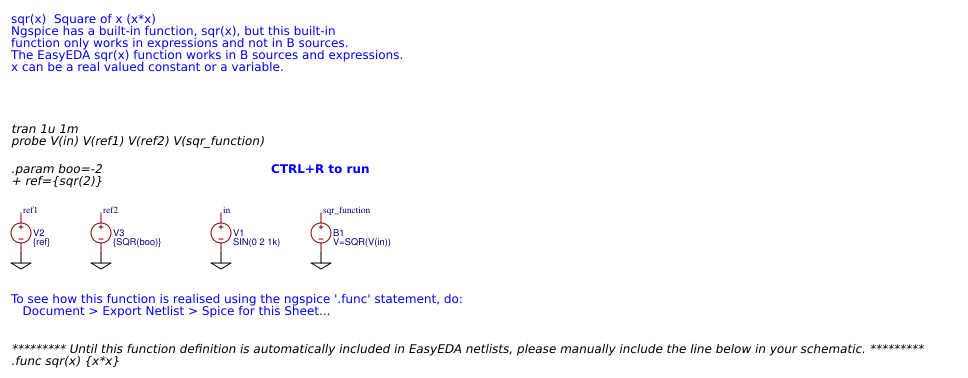## sqrt(x)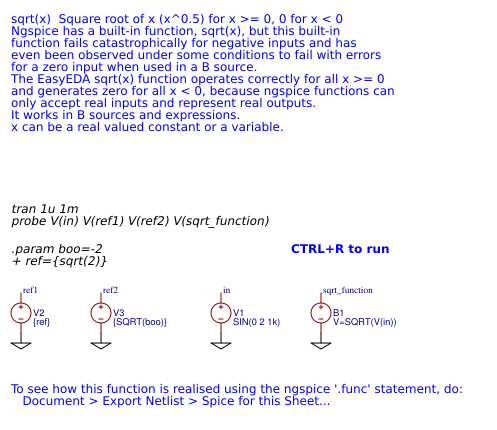## exp(x)## invsin(x)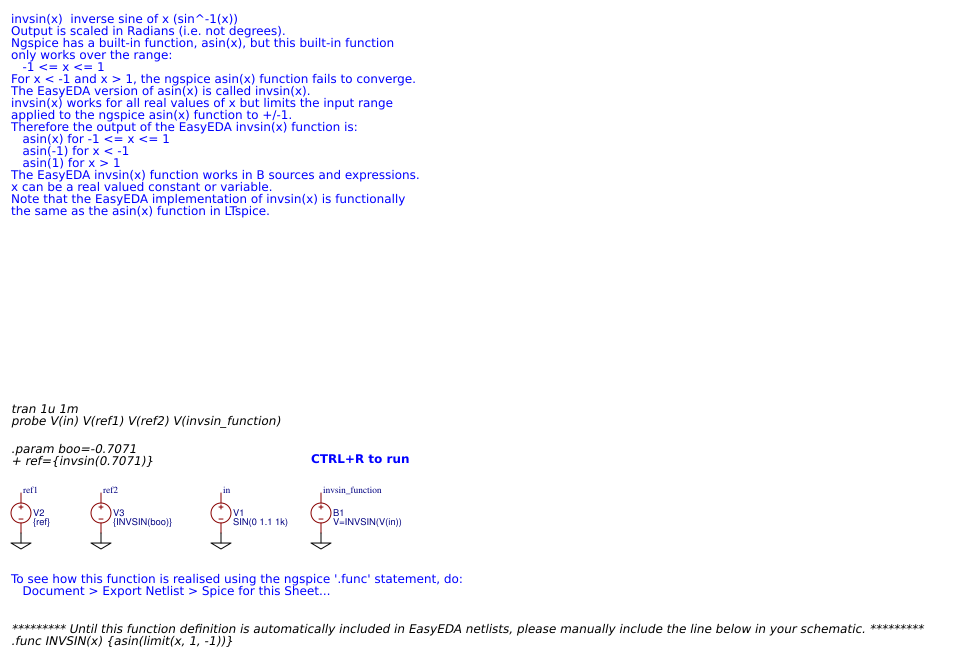## invcos(x)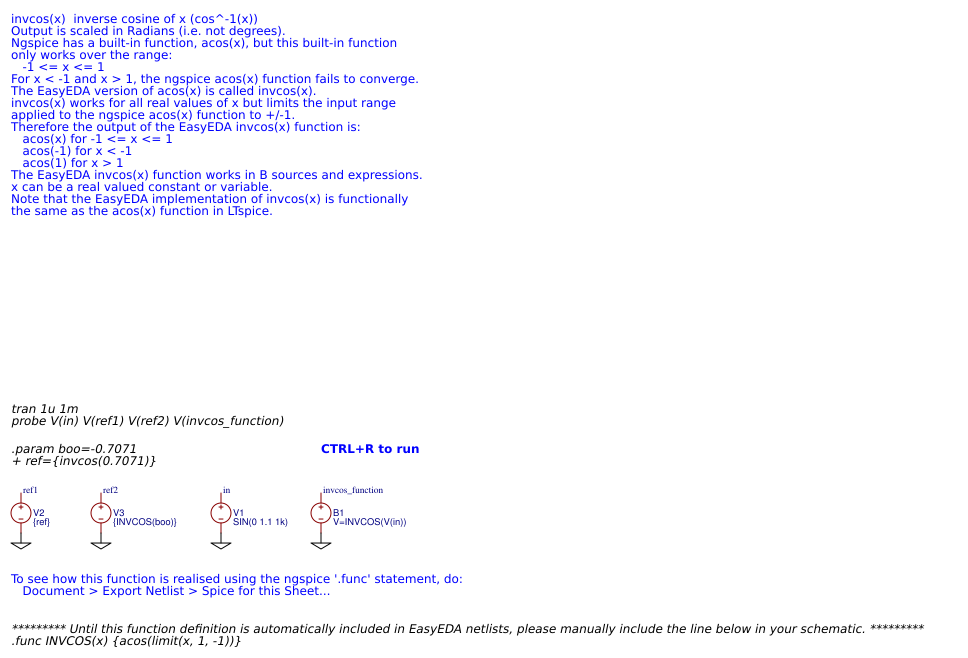## atan(x) arctan(x)## sin(x)## atan2(y,x)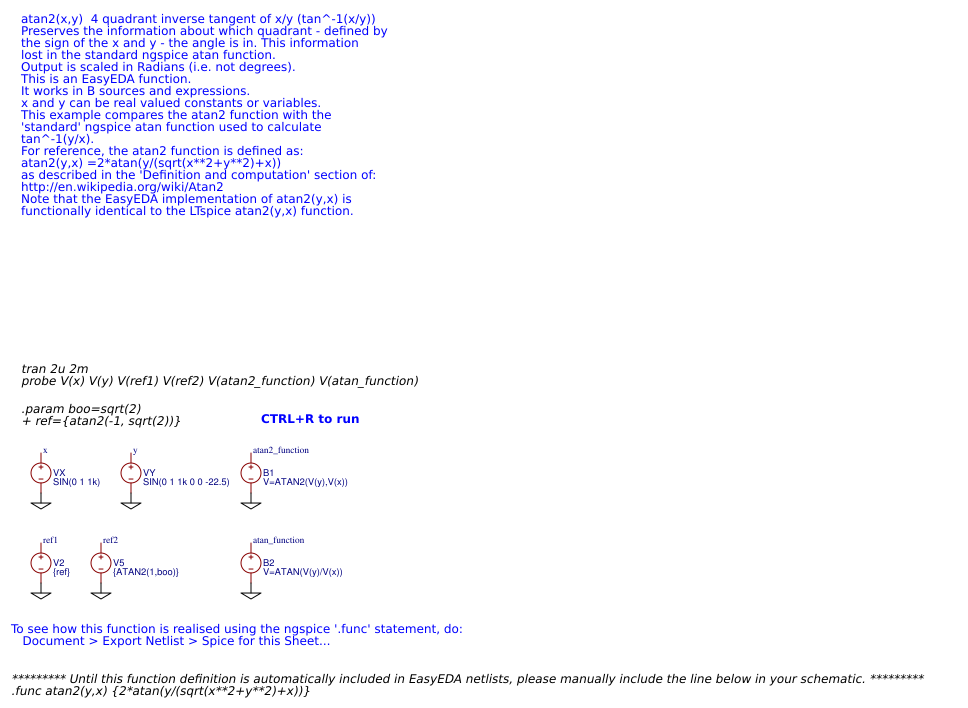## cos(x)## tan(x)## asin(x)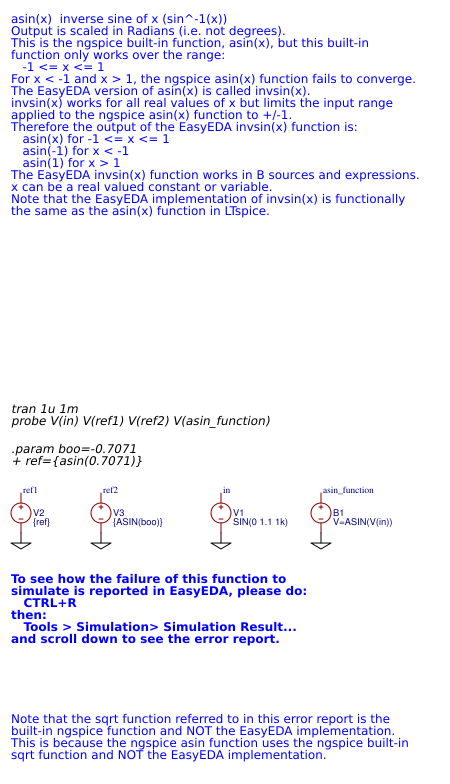## acos(x)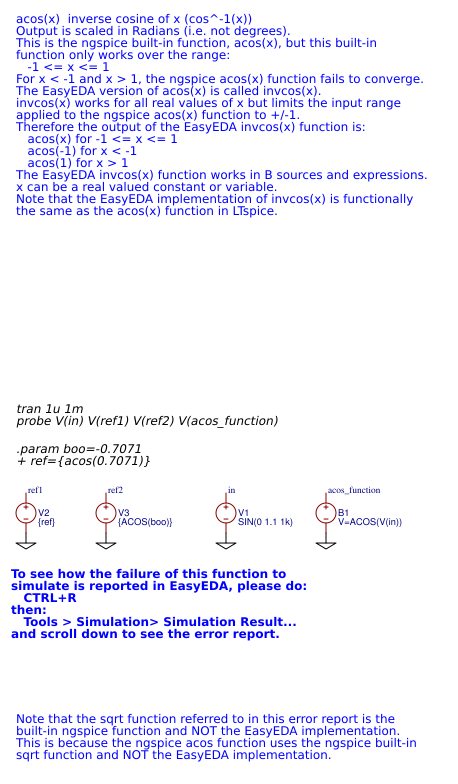## sgn(x)## cosh(x)## invtan(x)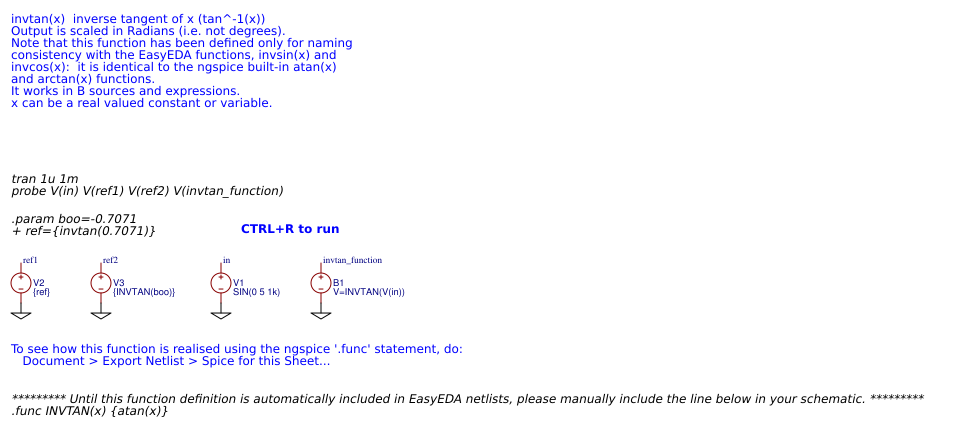## tanh(x)## sinh(x)## atanh(x)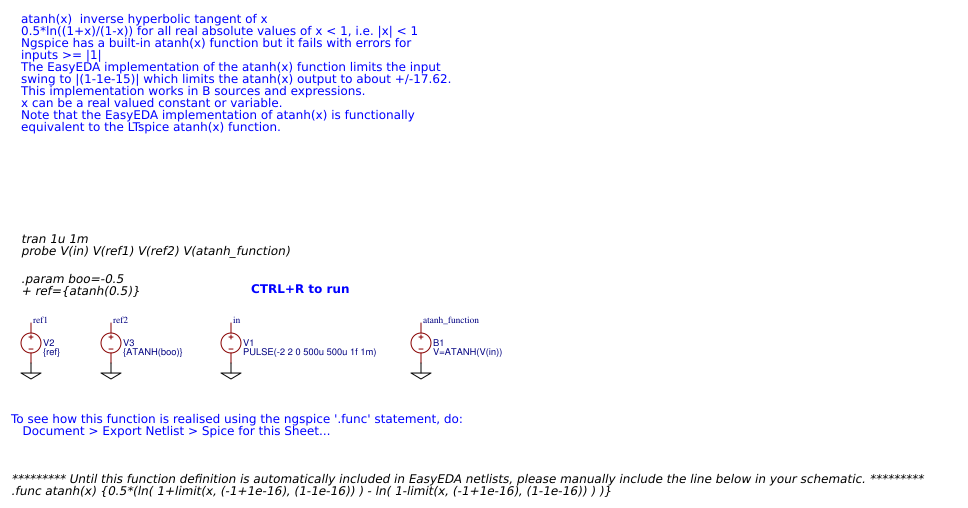## acosh(x)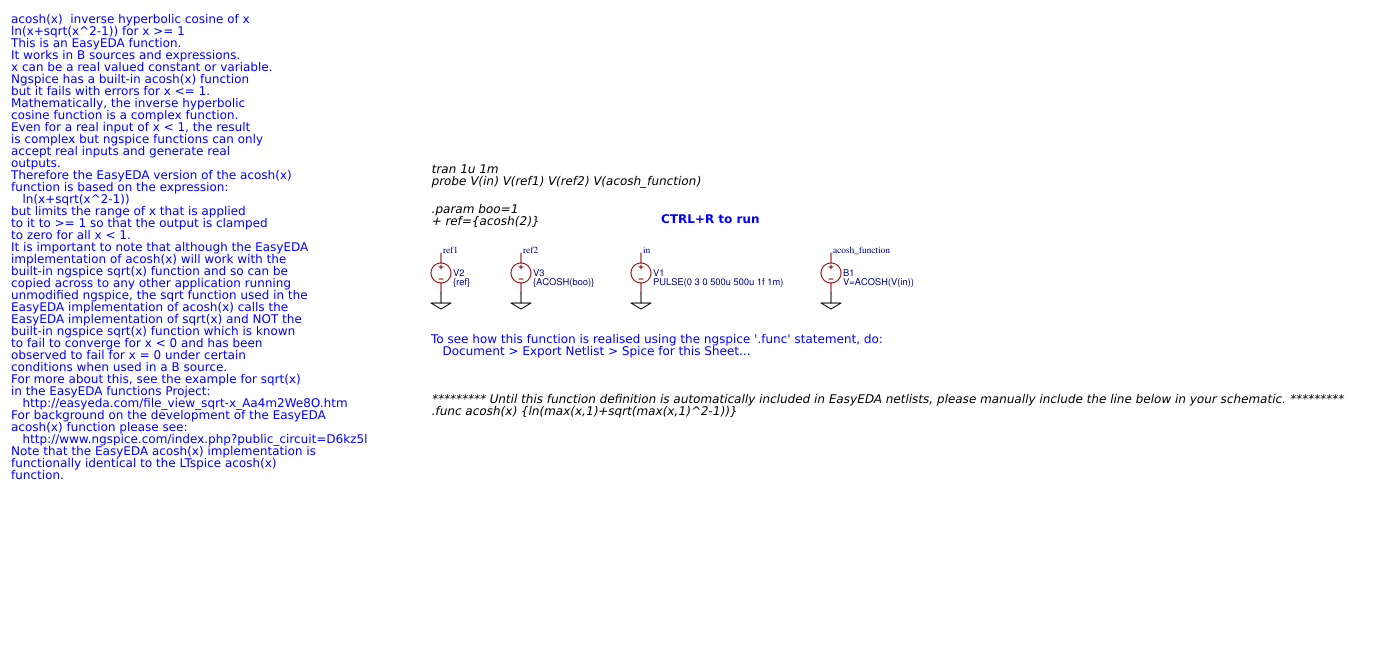## asinh(x)## ifx(x,y,z)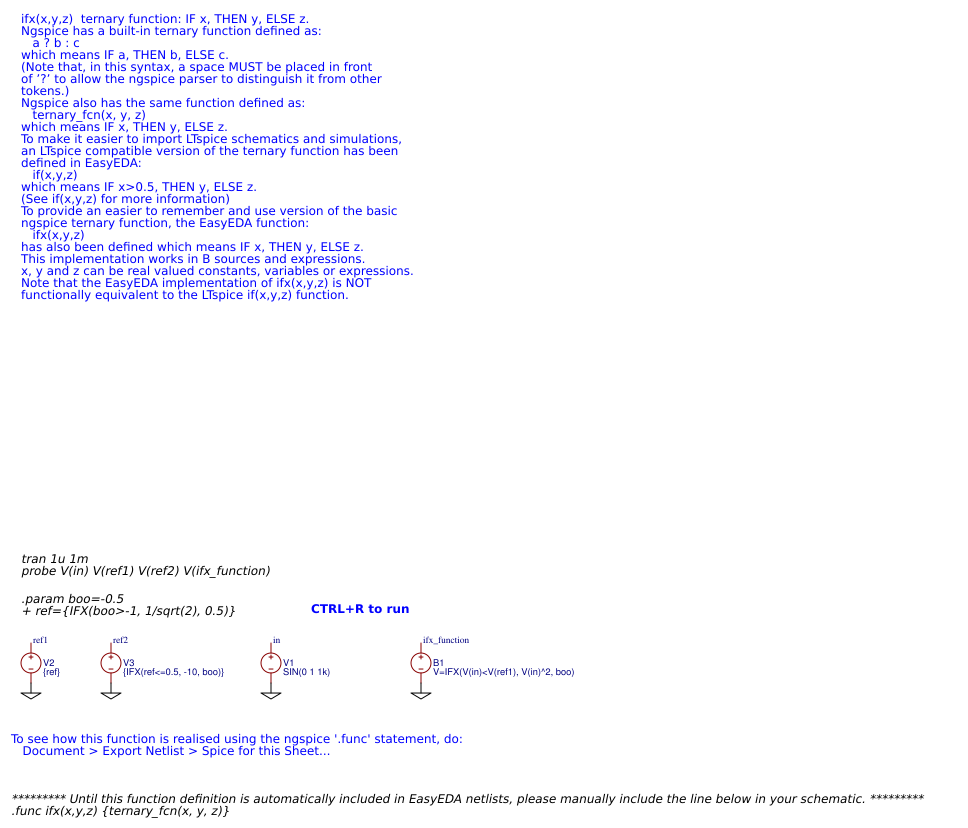## if(x,y,z)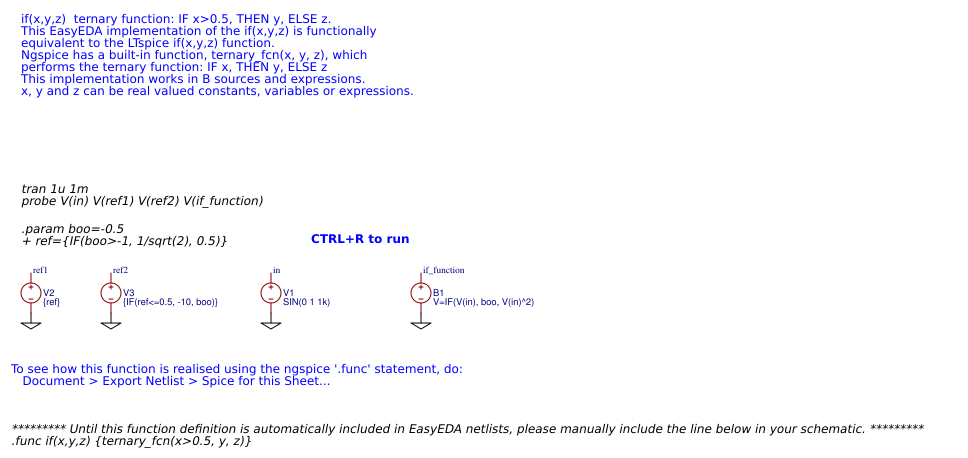## log(x)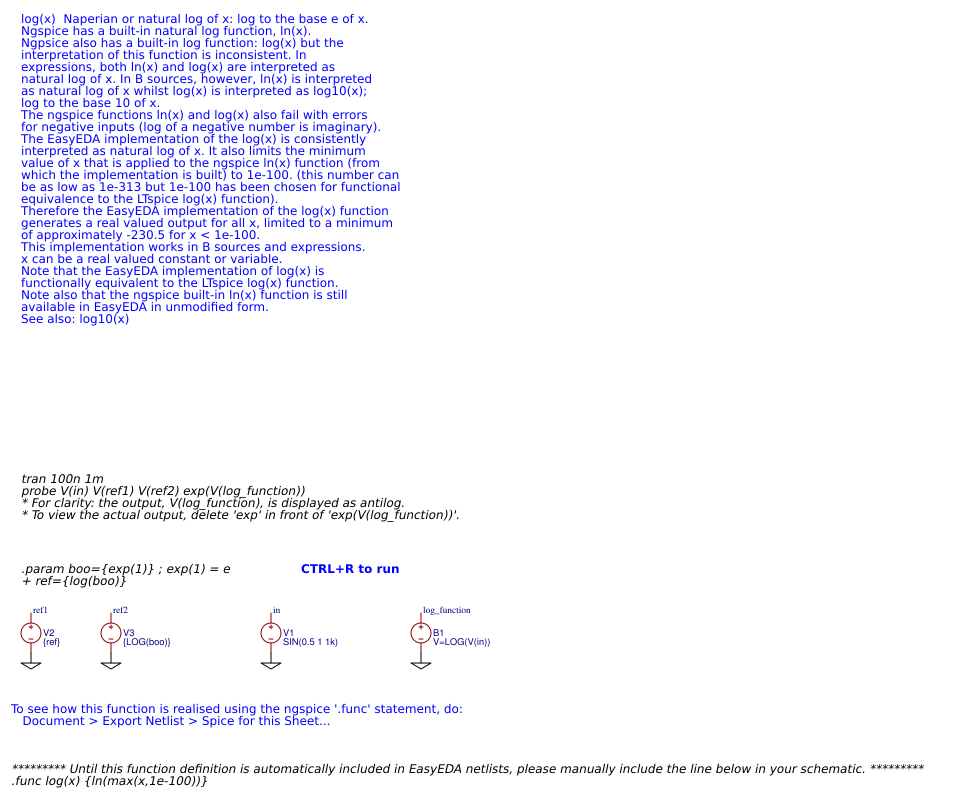## ln(x)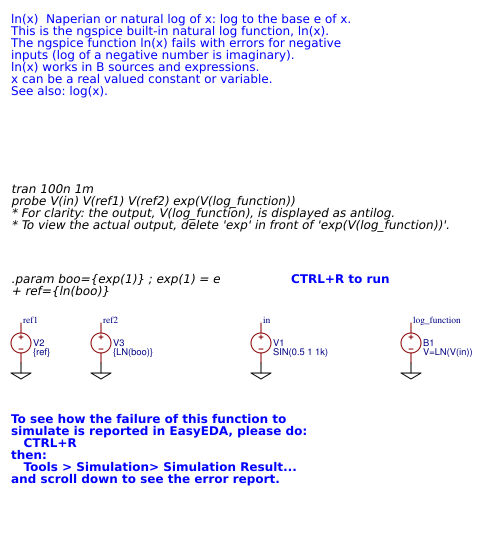## log10(x)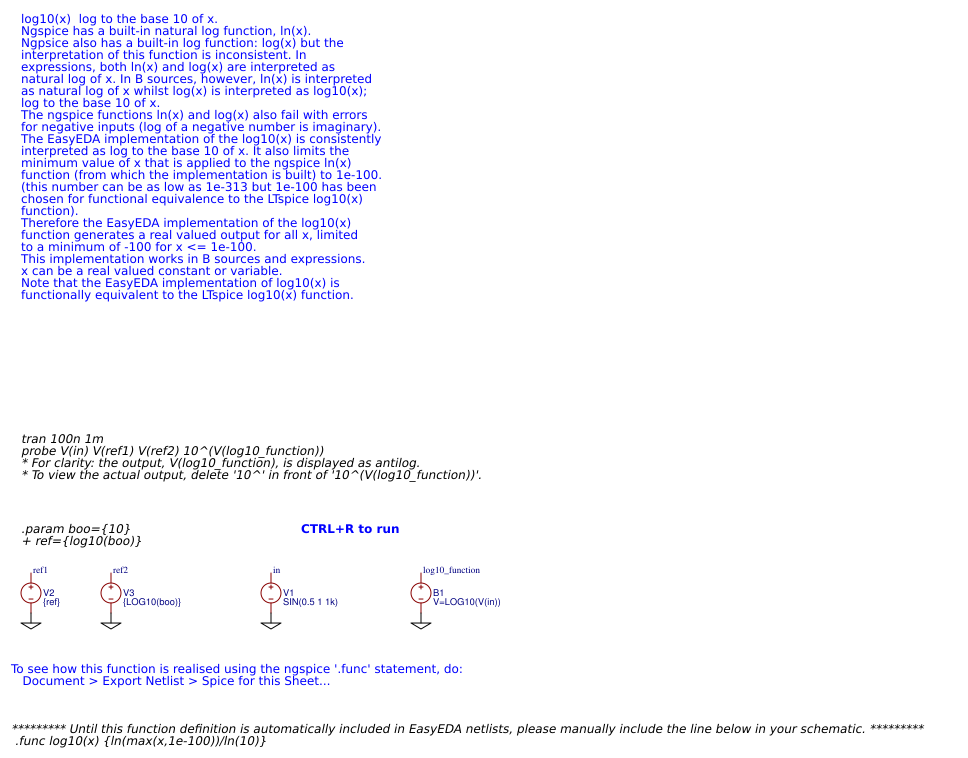## u(x), stp(x)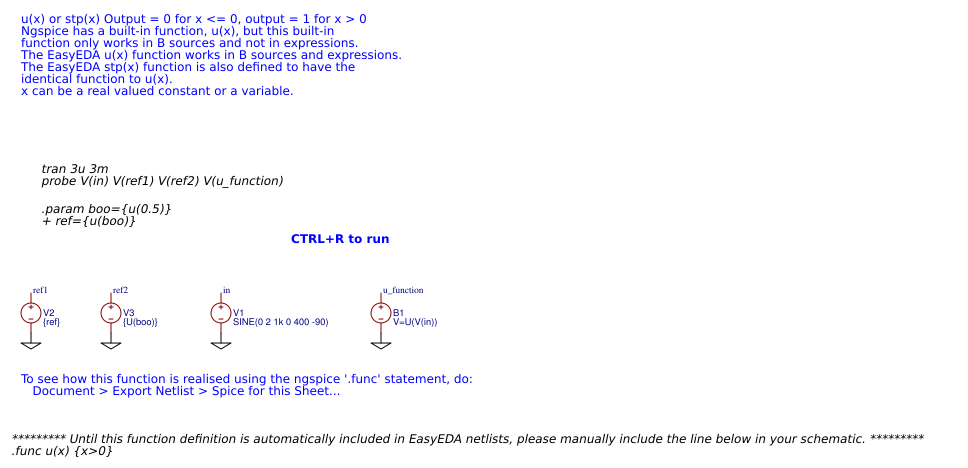## u2(x)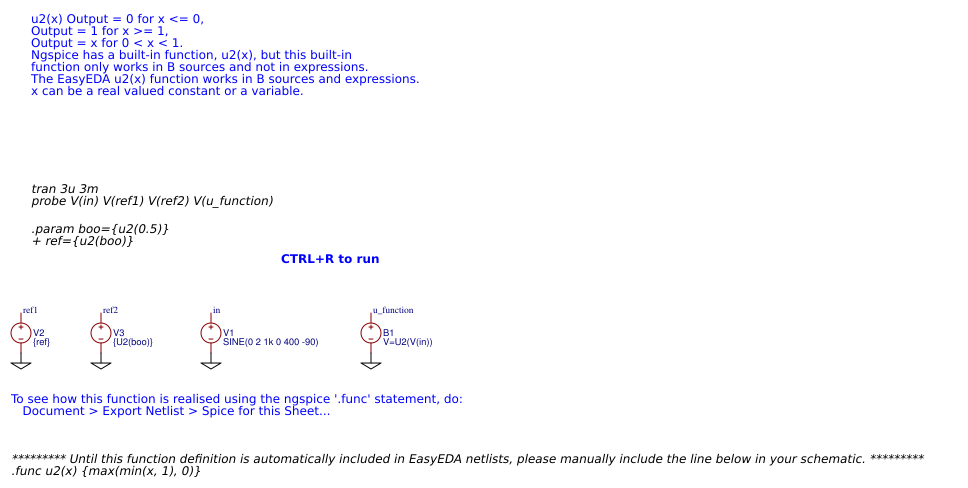## buf(x)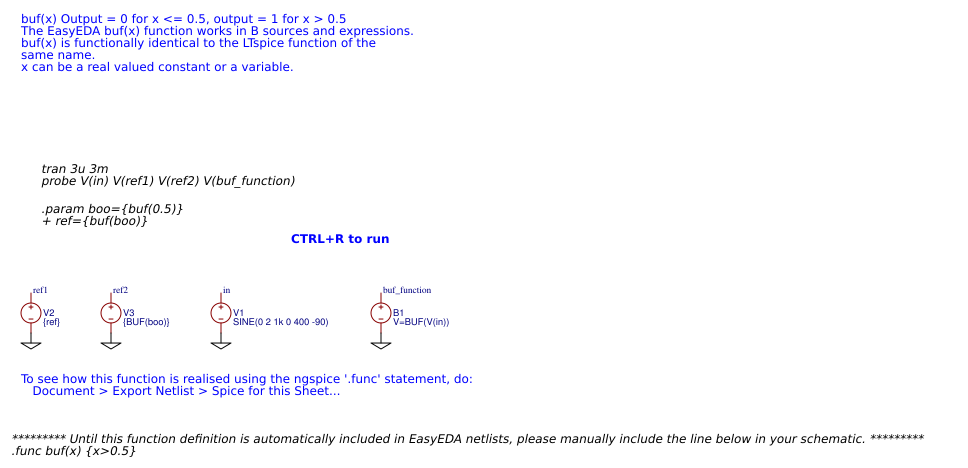## inv(x)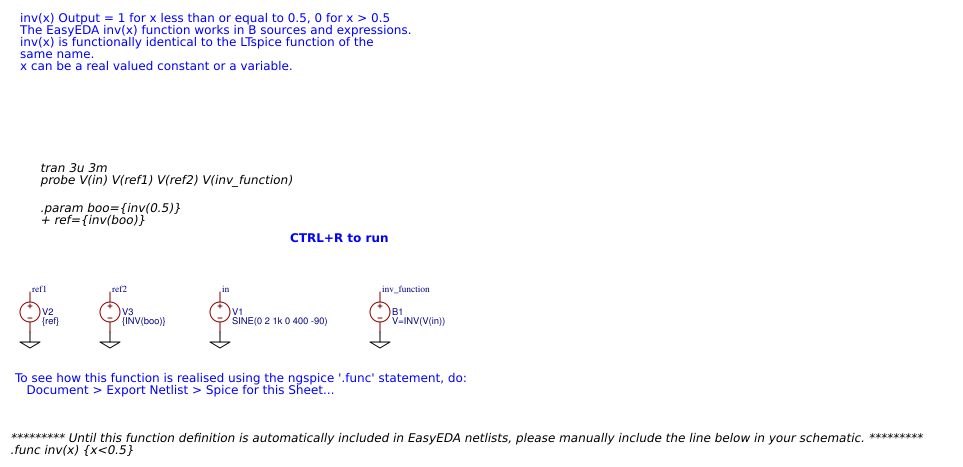## softlim(ip, lo, hi, sharp)None

## Attachments

None
Success
The owner does not allow comments in this project now